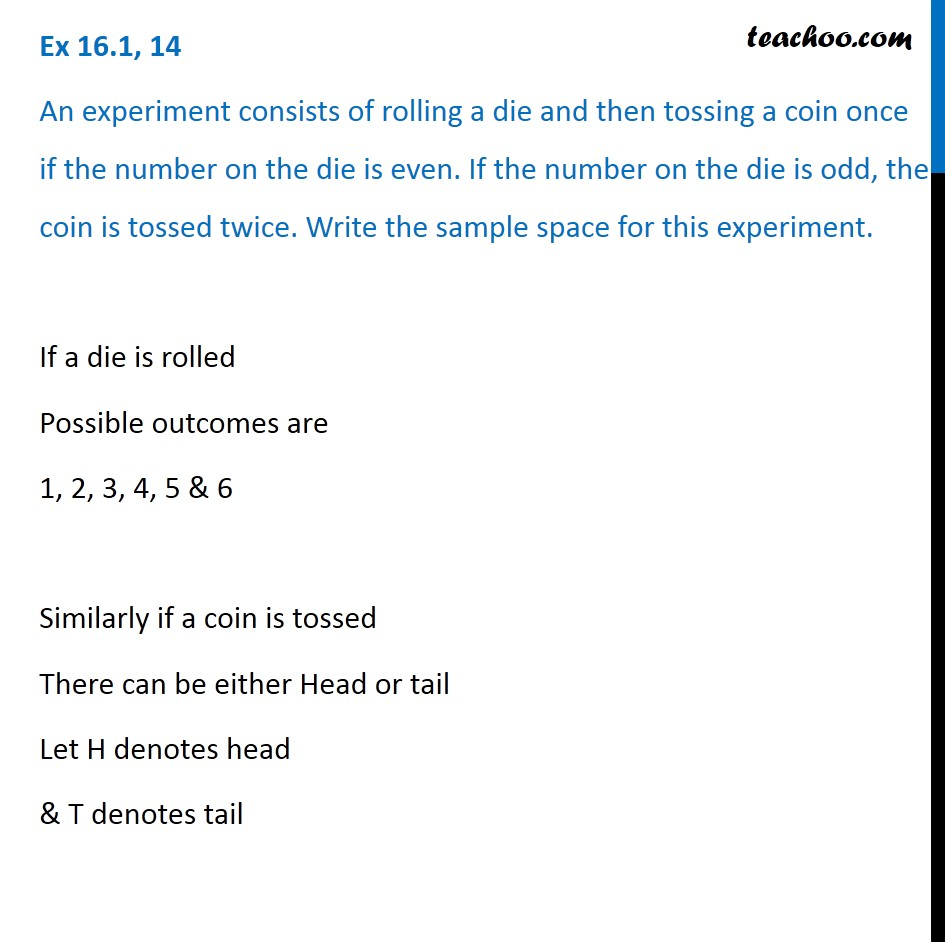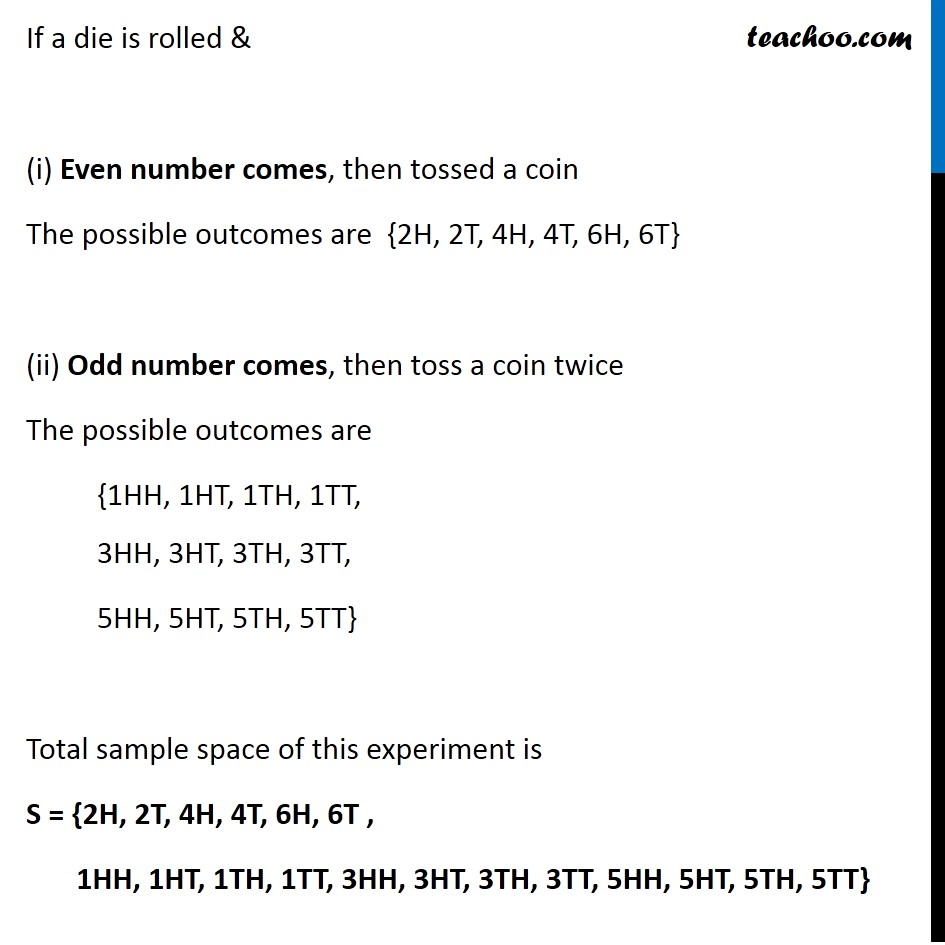1. Chapter 16 Class 11 Probability
2. Concept wise
3. Sample Space

Transcript

Ex 16.1, 14 An experiment consists of rolling a die and then tossing a coin once if the number on the die is even. If the number on the die is odd, the coin is tossed twice. Write the sample space for this experiment. If a die is rolled Possible outcomes are 1, 2, 3, 4, 5 & 6 Similarly if a coin is tossed There can be either Head or tail Let H denotes head & T denotes tail If a die is rolled & (i) Even number comes, then tossed a coin The possible outcomes are {2H, 2T, 4H, 4T, 6H, 6T} (ii) Odd number comes, then toss a coin twice The possible outcomes are {1HH, 1HT, 1TH, 1TT, 3HH, 3HT, 3TH, 3TT, 5HH, 5HT, 5TH, 5TT} Total sample space of this experiment is S = {2H, 2T, 4H, 4T, 6H, 6T , 1HH, 1HT, 1TH, 1TT, 3HH, 3HT, 3TH, 3TT, 5HH, 5HT, 5TH, 5TT}

Sample Space

About the AuthorDavneet Singh
Davneet Singh is a graduate from Indian Institute of Technology, Kanpur. He has been teaching from the past 10 years. He provides courses for Maths and Science at Teachoo.